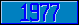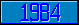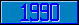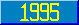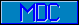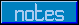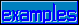## GKS - Principles

### gksdat

Introduced in the 1994 ANSI GKS - M[UMPS] language binding.

This metalanguage symbol represents a data record. This entity can be any expression.

### gksenum

Introduced in the 1994 ANSI GKS - M[UMPS] language binding.

This metalanguage symbol represents an entity that has one out of a number of possible values (an enumerated list of possible values). This entity can be any expression.

### gksfile

Introduced in the 1994 ANSI GKS - M[UMPS] language binding.

This metalanguage symbol represents the name of a file. This entity can be any expression.

### gksint

Introduced in the 1994 ANSI GKS - M[UMPS] language binding.

This metalanguage symbol represents an integer number that is bounded by constraints within the GKS standard.This entity can be the integer interpretation of any expression.

### gksnam

Introduced in the 1994 ANSI GKS - M[UMPS] language binding.

This metalanguage symbol represents a name that matches constraints as specified within the GKS standard. This entity can be any expression.

### gksnum

Introduced in the 1994 ANSI GKS - M[UMPS] language binding.

This metalanguage symbol represents a number that is bounded by constraints within the GKS standard.This entity can be the numeric interpretation of any expression.

### gksplst

Introduced in the 1994 ANSI GKS - M[UMPS] language binding.

This metalanguage symbol represents a list of points (separated by commas). A point is represented by a list of integer numbers (separated by commas). Since the GKS processor knows which type of coordinates is being used (two-dimensional or three-dimensional), there is no ambiguity about which commas separate coordinates, and which commas separate points.

### gkspnt

Introduced in the 1994 ANSI GKS - M[UMPS] language binding.

This metalanguage symbol represents a point. A point is represented by a list of integer numbers (separated by commas). The number of values depends on the projection space (two-dimensional, three-dimensional) that is currently being used.

### gksstr

Introduced in the 1994 ANSI GKS - M[UMPS] language binding.

This metalanguage symbol represents an expression; the value of this expression is not restricted to be anything specific.

### M

Introduced in the 1994 ANSI GKS - M[UMPS] language binding.

This metalanguage symbol represents an array of which the elements meet a certain constraint.

v1 ::= M gksnam means: an array v1(1),v1(2),v1(3), ... of which the elements each contain a value that evaluates to a name that meets the constraints specified in the GKS standard.